Formatting of Strings in Julia

• Last Updated : 25 Aug, 2020

Julia is a high-level language developed by people at Massachusetts Institute of Technology. It is an open-source, high-performance language. It has a simple syntax like Python but has speed and performance of C.

String formatting is the process of evaluating a string literal using string interpolation (filling unknown values between known values), it yields a result in which placeholders are replaced with their corresponding values.

For example:

word="article"
@printf("This is an %s",word)

The upper code will replace ‘%s’ with the value of word and the output will be:

This is an article

Most current languages provide different types of format specifiers and different formatting functions as well. In Julia, there are format specifiers somewhat similar to C:

• %c: a single character (letter, number, special symbol)
• %s: string (a combination of characters)
• %d: integer (any whole number (not a fraction))
• %f: float (numbers having floating decimal points)
• %.nf: float restricted up to n decimal places
• %e: scientific representation of a float number

There are 2 ways to format a string in Julia.

Method 1: Using Printf Library

Printf is a standard library in Julia that is included by following the syntax:

using Printf

Julia uses printf() function similar to C, in order to format strings. Here, we print using the macro @printf.

Printing different data types

Characters: In the following code, we are storing a single letter ‘c’ in the variable named ‘character’. The character variable is then printed using ‘%c’ format specifier as its data type is char. To make the specifier functionality more understandable another variable ‘character2’ with the same data type (character) is used.

Julia

 using printfcharacter = 'c';@printf("the letter is %c", character);character2 = 'a';@printf("the two letters are %c and %c",                 character, character2);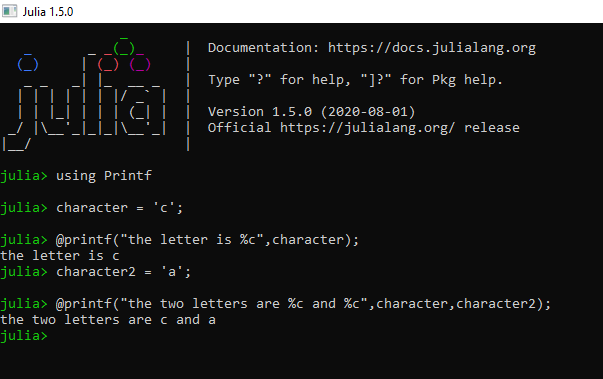In the output, it can be clearly seen that %c is replaced with the value of the character variable in the first printf statement and both %c are replaced with values of character and character2 in the second printf statement.

Strings: In the following example a string ‘GeeksforGeeks’ is stored in a variable ‘s’ and is printed using the ‘%s’ specifier and printf. To make this code more understandable, another string S2 is added whose value is ‘I love’, and both the strings are printed together making it ‘I love GeeksforGeeks’.

Julia

 # Loading Libraryusing Printf  # Creating Strings = "GeeksforGeeks";  # Formatting first string@printf("welcome to %s", s);  # Creating second strings2 = "I love";  # Formatting both strings@printf("%s %s", s2, s);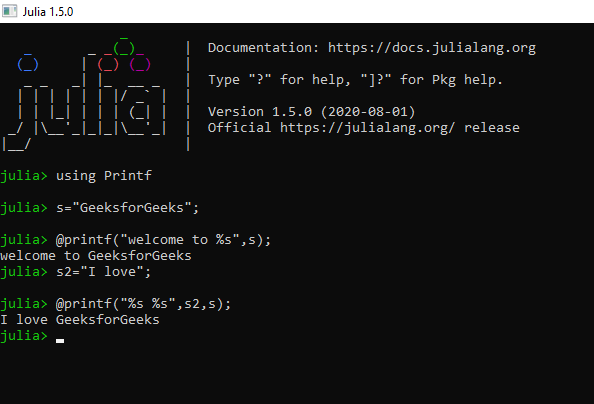In the output, you can see how in the first printf statement %s is replaced with the value of s. Similarly, in the second printf statement, both %s are replaced with s2 and s.

Integers: In the code below, we have defined a variable named integer as 33. We have then printed it using ‘%d’ specifier. Similarly, we have defined one more variable integer2 and have set its value to 42. In the second printf statement, we have printed the sum of both these numbers.

Julia

 using Printfinteger = 33;@printf("the value is %d",integer);integer2 = 42;@printf("the sum is %d + %d = %d",                integer, integer2,                 integer + integer2);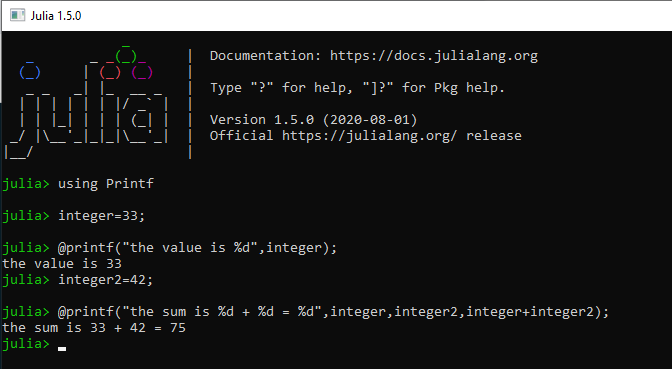In the output, look at how ‘%d’ is replaced with the value of integer in the first printf statement. And, in the second printf statement %d,%d and %d are replaced with integer, integer2, and integer+integer2.

Float: In the code below, we have declared a variable ‘floatvalue’ and set it to 0.08. We have then printed this value using ‘%f’ specifier. Then we initialized one more variable ‘floatvalue2’ and set it to 1.92. In the second printf statement, we have printed sum of both these values using specifiers ‘%d’ and ‘%f’.

Julia

 using Printffloatvalue = 0.08;@printf("the floating point value is %f",                              floatvalue);floatvalue2 = 1.92;@printf("the value of %f + %f = %d",             floatvalue, floatvalue2,            floatvalue + floatvalue2);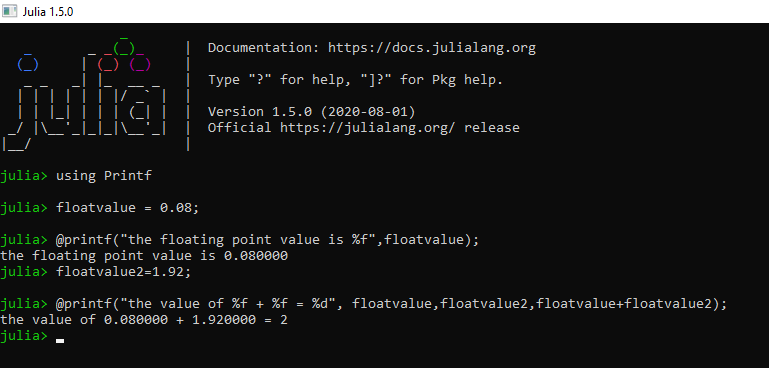You can see how %f is replaced with 0.08 in the first output and %f,%f, and %d are replaced with 0.08, 1.92, and 2 respectively. (Notice how we used ‘%d’ to print this sum and obtained integral value of the sum if you wish to obtain the float value of sum you can use ‘%f’ specifier.)

Floating-point values restricted to 2 decimal places: Suppose we have the value of pi and we want to display it, but instead of displaying its whole value we just want to print the value ‘3.14’ i.e. the value should be restricted to 2 decimal places. For this we use %.nf (n being the limit).

Julia

 using Printffloatvalue = 20.3658;@printf("the value of float restricted to 2 decimal places %.2f",                                                     floatvalue);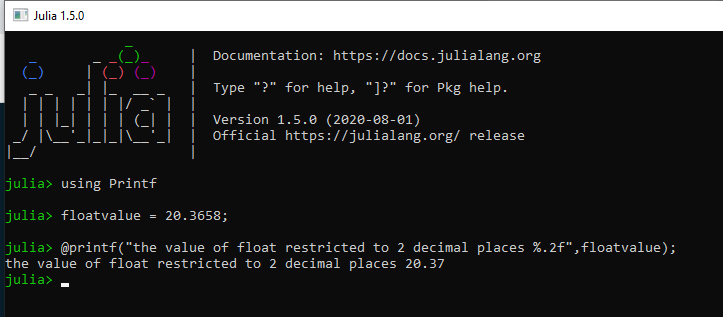In this output, you can see how 20.3658 is printed as 20.37 as it is rounded up to 2 decimal places.

Scientific representation of a floating-point number: You can print out the scientific representation of a floating-point number using ‘%e’ specifier. It is usually used to represent very large or very small numbers.

Julia

 using Printffloatvalue = 20.3658;@printf("scientific representation of the floatvalue is %e",                                                floatvalue);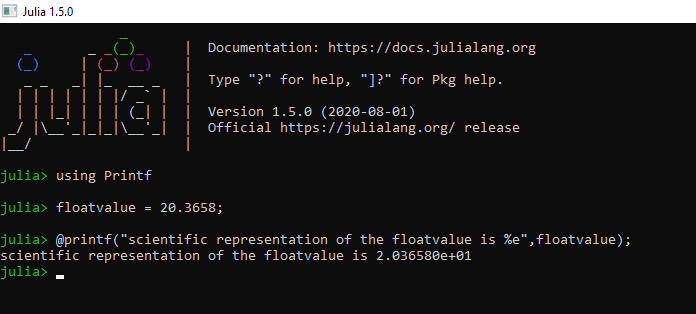In this output, notice how ‘floatvalue’ (20.3658) is displayed as 2.063580e+01. This happened because we used ‘%e’ specifier and it printed out its scientific representation.

Method 2: Using Formatting.jl Package

Formatting.jl is an open-source Julia package that was made to provide python like formatting to Julia. This package is available on Github Formatting.jl.

We can use printfmt or printfmtln for formatted printing. Additionally, “fmt” and “format” can be used to format string values.

Example: Printing different data types

In this method, formatting is done similarly to that in python. Format specifiers are put inside curly braces {} and ‘%’ symbol is replaced with ‘:’  (look in the code below).

Julia

 using Formatting  # to print a stringsite = "GeeksforGeeks";printfmt("Welcome to {:s}", site)  # to print a single characterc = 'a';printfmt("Character is {:c}", c)  # to print an integernum = 40;printfmt("Integer is {:d}", num)  # to print a float valuefloatnum = 178.33987;printfmt("Float value is {:f}", floatnum)  # to print a float value # restricted to 2 decimal placesprintfmt("Restricted to 2 decimal places {:.2f}",                                        floatnum)  # to print a float value # in scientific representationprintfmt("Scientific representation {:e}",                                 floatnum)

Output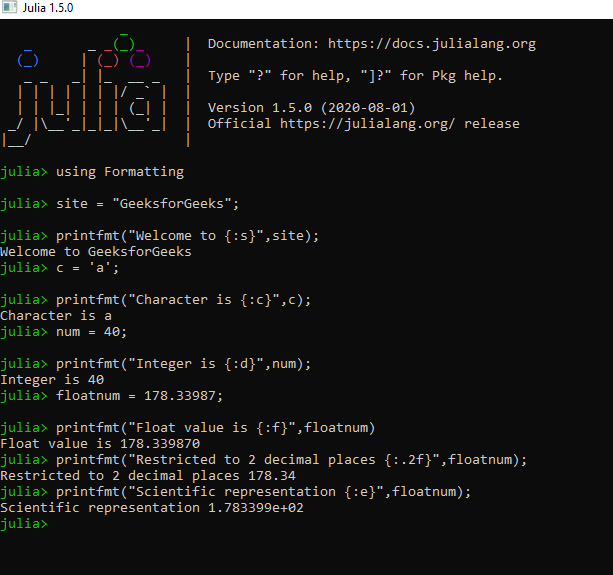In the output, you can see how {:c}, {:s}, {:d}, {:f}, {:e}, {:.2f}, are replaced with characters, strings, integers, floating-point numbers, scientific representations and floating point numbers restricted to 2 digits after decimal, respectively.

My Personal Notes arrow_drop_up